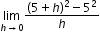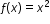# MA.912.C.2.1

State, understand and apply the definition of derivative. Apply and interpret derivatives geometrically and numerically.

### Examples

Example: Find. What does the result tell you? Use the limit to determine the derivative function for.
General Information
Subject Area: Mathematics (B.E.S.T.)
Strand: Calculus
Status: State Board Approved

## Related Courses

This benchmark is part of these courses.
1202300: Calculus Honors (Specifically in versions: 2014 - 2015, 2015 - 2022, 2022 and beyond (current))

## Related Access Points

Alternate version of this benchmark for students with significant cognitive disabilities.

## Related Resources

Vetted resources educators can use to teach the concepts and skills in this benchmark.

## Tutorials

Calculus: Derivatives 1:

In this video we will learn through an example, that a derivative is simply the slope of a curve at any given point.

Type: Tutorial

Calculating Slope of Tangent Line Using Derivative Definition:

In this video we will find the slope of the tangent line using the formal definition of derivative.

Type: Tutorial

Derivative as Slope of a Tangent Line:

We will find the derivative of a function by finding the slope of the tangent line.

Type: Tutorial

Mean Value Theorem:

In this video we will take an in depth look at the Mean Value Theorem.

Type: Tutorial

The Derivative of f(x)=x^2 for Any x:

In this video we will find the derivative of a function based on the slope of the tangent line.

Type: Tutorial

Chain Rule Example Using Visual Information:

In this video we will analyze the graph of a function and its tangent line, then use the chain rule to find the value of the derivative at that point.

Type: Tutorial

## Student Resources

Vetted resources students can use to learn the concepts and skills in this benchmark.

## Tutorials

Calculus: Derivatives 1:

In this video we will learn through an example, that a derivative is simply the slope of a curve at any given point.

Type: Tutorial

Calculating Slope of Tangent Line Using Derivative Definition:

In this video we will find the slope of the tangent line using the formal definition of derivative.

Type: Tutorial

Derivative as Slope of a Tangent Line:

We will find the derivative of a function by finding the slope of the tangent line.

Type: Tutorial

Mean Value Theorem:

In this video we will take an in depth look at the Mean Value Theorem.

Type: Tutorial

The Derivative of f(x)=x^2 for Any x:

In this video we will find the derivative of a function based on the slope of the tangent line.

Type: Tutorial

Chain Rule Example Using Visual Information:

In this video we will analyze the graph of a function and its tangent line, then use the chain rule to find the value of the derivative at that point.

Type: Tutorial

## Parent Resources

Vetted resources caregivers can use to help students learn the concepts and skills in this benchmark.